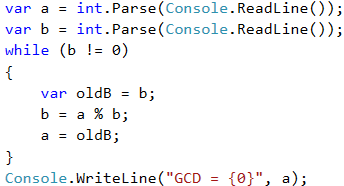# Greatest Common Divisor (GCD)

Before proceeding to the next problem, we will get familiar with the definition of the greatest common divisor (GCD), widely used in mathematics and numbers theory, and will learn how to calculate GCD.

Definition of GCD: the greatest common divisor of two natural numbers a and b is the largest number that divides both a and b without reminder.

a b GCD
24 16 8
67 18 1
12 24 12
15 9 3
10 10 10
100 88 4

## Video: Greatest Common Divisor (GCD)

Watch the video lesson to learn about the Euclidean algorithm for calculating the GCD of given two integers: https://youtu.be/1-SEOWupvrA.

## The Euclidean Algorithm

In the next problem we will use one of the first published algorithms for finding the GCD – Euclid's algorithm.

Until we reach a remainder of 0:

• We divide the greater number by the smaller one.
• We take the remainder of the division.

Euclid's algorithm pseudo-code:

while b ≠ 0
var oldB = b;
b = a % b;
a = oldB;
print a;


## Example: Greatest Common Divisor (GCD)

Enter integers a and b and find GCD(a, b).

We will solve the problem through Euclid's algorithm:

• We create variables a and b to which we assign integer values taken from the console input.
• For a loop condition, we put an expression that is true if the number b is different from 0.
• In the body of the loop we follow the instructions from the pseudo code:
• We create a temporary variable to which we assign the current value of b.
• We assign a new value to b, which is the remainder of the division of a and b.
• On the variable a we assign the previous value of the variable b.
• Once the loop is complete and we have found the GCD, we print it on the screen.

This is a sample implementation of the Euclidean algorithm: